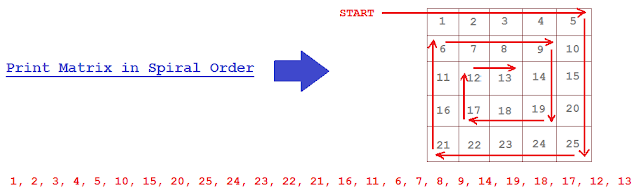# Print Matrix in Spiral order using Recursion.

Print Matrix in Spiral order OR
Given m*n matrix(m rows, n columns), print all elements of the matrix in spiral order OR

Print two-dimensional array in spiral order OR
How to print elements of Matrix in Spiral Format?

Given a Matrix(2D array), print it in spiral form. Lets understand the problem statement graphically and it will be more clear,### Algorithm

There are 2 ways to print matrix in Spiral order.
1. Iterative.
2. Recursive.

### We already saw Iterative approach: Print matrix in spiral order In this post, we will focus on Recursive approach.

In Iterative approach, we need to maintain 4 variables rowStart, rowLength, colStart, colLength which help in printing matrix in Spiral way.

1. Left to Right.
Move variable i from rowStart till colLength. (
Print data from first row till last column.)

2. Top to Bottom.
Move variable i from (rowStart+1) till rowLength. (Print data in last column till)
We need to start from
(rowStart+1) because, we already printed corner element in Left to Right printing and no need to include it again. Same treatment for corner elements in other directions.

3. Right to Left.
Move variable i from colLength-1 till colStart. (Print data in last row)

4. Bottom to Up.
Move variable i from rowLength-1 till rowStart. (Print data in first column)

5. After printing all 4 directions, In next iteration,
we need to start from second row and second column, so increment
(rowStart ++) and (colStart++).

we need to print till second Last column and till second Last row, so decrement
(colLength --) and
(rowLength --).

In Recursive approach, we will be requiring same 4 variable and follow the same process, only difference in this approach will be, after printing first outer Layer of Matrix elements, before moving into inner layers of Matrix, we will check whether next inner layer of Matrix is present or this is end of all Layers.

If Matrix has inner Layer present, we will increment rowStart++, colStart++ and decrement rowLength--, colLength--, which now points to inner matrix layer and pass this variable to next recursive call.

### Java Program to Print Matrix in Spiral order using Recursion

```package matrix;

public class PrintMatrixInSpiralForm {

public static void main(String[] args) {
new PrintMatrixInSpiralForm();
}

public PrintMatrixInSpiralForm() {
int[][] matrix = {
{1,4,7,10},
{2,5,8,11}
};

printMatrixInSpiralWayUsingRecursion(matrix,0,0,matrix.length-1,matrix.length-1);
}

private void printMatrixInSpiralWayUsingRecursion(int[][] matrix, int rowStart, int colStart, int colLength,  int rowLength){
for (int i = rowStart; i <= colLength; i++) {
System.out.print(matrix[rowStart][i] + " ");
}
for (int i = rowStart+1; i <= rowLength; i++) {
System.out.print(matrix[i][colLength] + " ");
}

if(rowStart+1 <= rowLength){
for (int i = colLength-1; i >= colStart; i--) {
System.out.print(matrix[rowLength][i] + " ");
}
}

if(colStart+1 <= colLength){
for (int i = rowLength-1; i > rowStart; i--) {
System.out.print(matrix[i][colStart] + " ");
}
}

if(rowStart+1 <= rowLength-1 && colStart+1 <= colLength-1){
printMatrixInSpiralWayUsingRecursion(matrix, rowStart+1, colStart+1, colLength-1, rowLength-1);
}
}
}

```

### You may also like to see

#### How is ambiguous overloaded method call resolved in java

Enjoy !!!!

If you find any issue in post or face any error while implementing, Please comment.